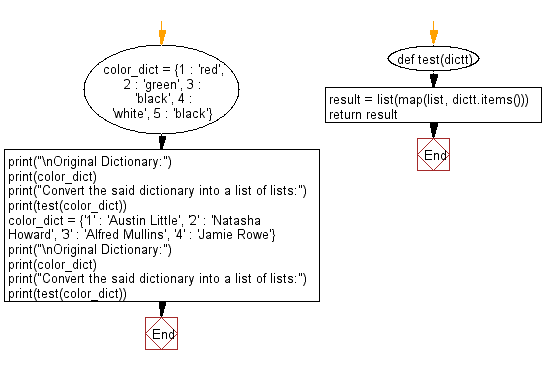﻿ Python: Convert a given dictionary into a list of lists - w3resource# Python: Convert a given dictionary into a list of lists

## Python dictionary: Exercise-56 with Solution

Write a Python program to convert a given dictionary into a list of lists.

Sample Solution:

Python Code:

``````def test(dictt):
result = list(map(list, dictt.items()))
return result

color_dict = {1 : 'red', 2 : 'green', 3 : 'black', 4 : 'white', 5 : 'black'}
print("\nOriginal Dictionary:")
print(color_dict)
print("Convert the said dictionary into a list of lists:")
print(test(color_dict))

color_dict = {'1' : 'Austin Little', '2' : 'Natasha Howard', '3' : 'Alfred Mullins', '4' : 'Jamie Rowe'}
print("\nOriginal Dictionary:")
print(color_dict)
print("Convert the said dictionary into a list of lists:")
print(test(color_dict))
```
```

Sample Output:

```Original Dictionary:
{1: 'red', 2: 'green', 3: 'black', 4: 'white', 5: 'black'}
Convert the said dictionary into a list of lists:
[[1, 'red'], [2, 'green'], [3, 'black'], [4, 'white'], [5, 'black']]

Original Dictionary:
{'1': 'Austin Little', '2': 'Natasha Howard', '3': 'Alfred Mullins', '4': 'Jamie Rowe'}
Convert the said dictionary into a list of lists:
[['1', 'Austin Little'], ['2', 'Natasha Howard'], ['3', 'Alfred Mullins'], ['4', 'Jamie Rowe']]
```

Flowchart:## Visualize Python code execution:

The following tool visualize what the computer is doing step-by-step as it executes the said program:

Python Code Editor:

Have another way to solve this solution? Contribute your code (and comments) through Disqus.

What is the difficulty level of this exercise?

Test your Programming skills with w3resource's quiz.

﻿

## Python: Tips of the Day

Clamps num within the inclusive range specified by the boundary values x and y:

Example:

```def tips_clamp_num(num,x,y):
return max(min(num, max(x, y)), min(x, y))
print(tips_clamp_num(2, 4, 6))
print(tips_clamp_num(1, -1, -6))
```

Output:

```4
-1
```